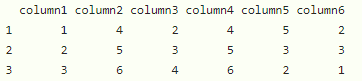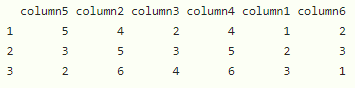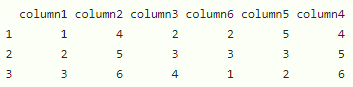Open in App
Not now

# How to Switch Two Columns in R DataFrame?

• Last Updated : 19 Dec, 2021

In this article, we will discuss how to switch two columns in dataframe in R Programming Language.

Let’s create the dataframe with 6 columns

## R

 `# create a dataframe ` `data = ``data.frame``(column1=``c``(1, 2, 3),  ` `                  ``column2=``c``(4, 5, 6),  ` `                  ``column3=``c``(2, 3, 4),  ` `                  ``column4=``c``(4, 5, 6),  ` `                  ``column5=``c``(5, 3, 2),  ` `                  ``column6=``c``(2, 3, 1)) ` ` `  `# display ` `data `

Output:## Method 1: Using column syntax

Here we have to specify the order of columns.

Syntax:

`data`

where data is the input dataframe

Example:

Exchange 1st column with 5th column.

## R

 `# dataframe ` `data = ``data.frame``(column1=``c``(1, 2, 3),  ` `                  ``column2=``c``(4, 5, 6),  ` `                  ``column3=``c``(2, 3, 4),  ` `                  ``column4=``c``(4, 5, 6),  ` `                  ``column5=``c``(5, 3, 2),  ` `                  ``column6=``c``(2, 3, 1)) ` ` `  `# display by exchanging first column  ` `# with 5th column ` `data[``c``(``"column5"``, ``"column2"``, ``"column3"``, ` `       ``"column4"``, ``"column1"``, ``"column6"``)] `

Output:## Method 2: Switch Two Columns Using Row and Column Syntax

Here we are going to specify a row also separated by a comma.

Syntax:

`data[,c("column1", "column2", "column3", "column4",...............)]`

where data is the input dataframe

Example:

Exchange 4th column with 6th column

## R

 `# dataframe ` `data=``data.frame``(column1=``c``(1,2,3), ` `                ``column2=``c``(4,5,6), ` `                ``column3=``c``(2,3,4), ` `                ``column4=``c``(4,5,6), ` `                ``column5=``c``(5,3,2), ` `                ``column6=``c``(2,3,1)) ` ` `  `# display by exchanging 4 th column  ` `# with 6 th column ` `data[,``c``(``"column1"``, ``"column2"``, ``"column3"``, ` `        ``"column6"``,``"column5"``,``"column4"``)]`

Output:My Personal Notes arrow_drop_up
Related Articles